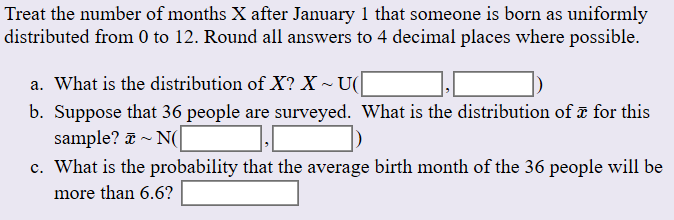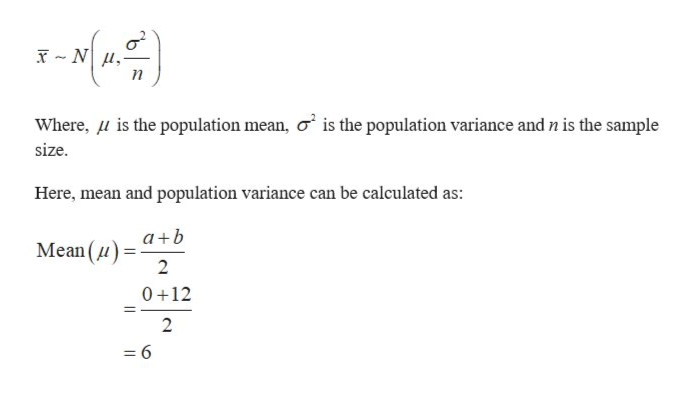# Treat the number of months X after January 1 that someone is born as uniformlydistributed from 0 to 12. Round all answers to 4 decimal places where possible.a. What is the distribution of X? XU(b. Suppose that 36 people are surveyed. What is the distribution of a for thissample? Nc. What is the probability that the average birth month of the 36 people will bemore than 6.6?

Question
387 viewshelp_outlineImage TranscriptioncloseTreat the number of months X after January 1 that someone is born as uniformly distributed from 0 to 12. Round all answers to 4 decimal places where possible. a. What is the distribution of X? X U( b. Suppose that 36 people are surveyed. What is the distribution of a for this sample? N c. What is the probability that the average birth month of the 36 people will be more than 6.6? fullscreen
check_circle

Step 1

According to the provided details, a = 0 and b = 12.

A uniform distribution has an equal probability for all the outcomes of the random variable, that is, it has a constant probability. A continuous uniform distribution is defined over the range, where a is the smallest value and b is the largest value of the distribution. The values, (a, b) are the parameters of the uniform distribution.

Step 2

(a)

Consider, X be the random variable which is uniformly distributed with the parameters a= 0 and b = 12, that is,

Step 3

(b)

Here, it is provided that the sample size (n) is 36.

The Central Limit Theorem states that if the sample size is greater than or equal to 30, the...help_outlineImage TranscriptioncloseWhere, u is the population mean, o is the population variance and n is the sample size. Here, mean and population variance can be calculated as: a+b Mean (u 2 0 12 2 = 6 fullscreen

### Want to see the full answer?

See Solution

#### Want to see this answer and more?

Solutions are written by subject experts who are available 24/7. Questions are typically answered within 1 hour.*

See Solution
*Response times may vary by subject and question.
Tagged in